Prime Factorization 3
Set 3 (10 Questions)
Determine the prime factors of all numbers through 50 and write the numbers as the product of their prime factors by using exponents to show multiples of a factor.
From Mr. Anker Tests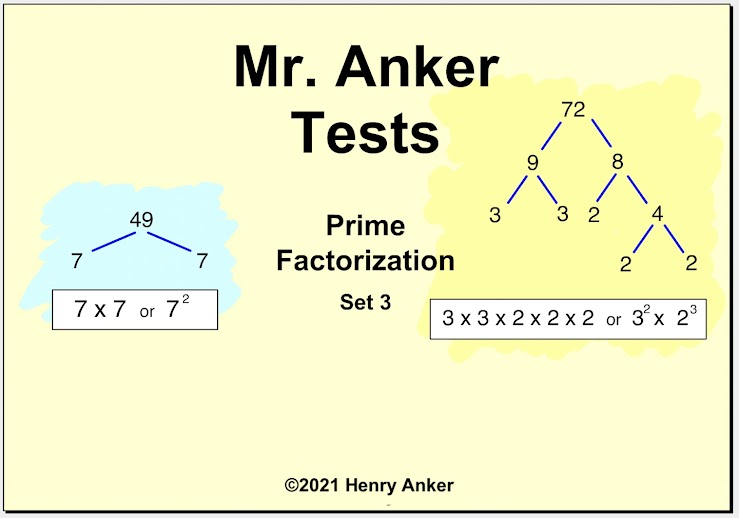Prime Factorization Help Slide 1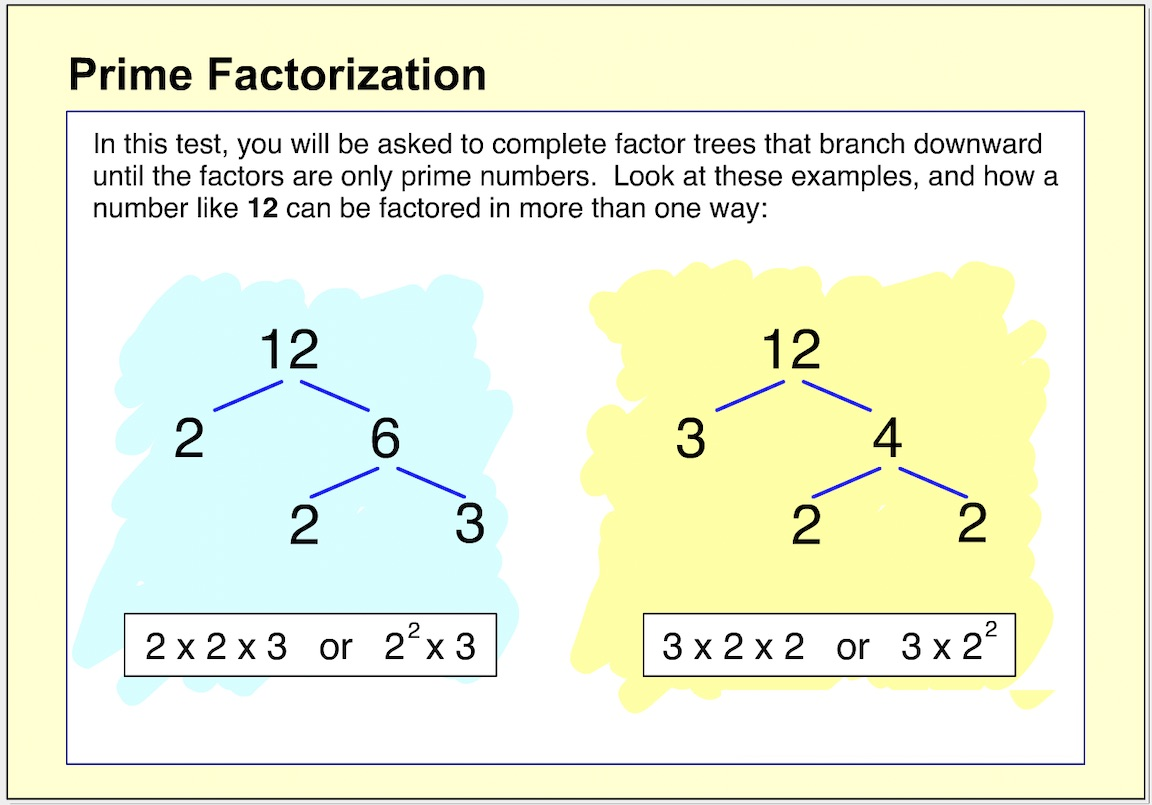Prime Factorization Help Slide 2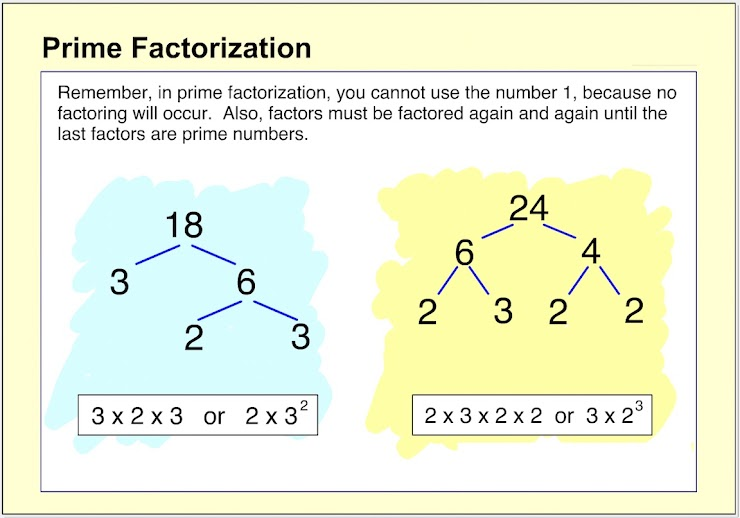Prime Factorization Help Slide 3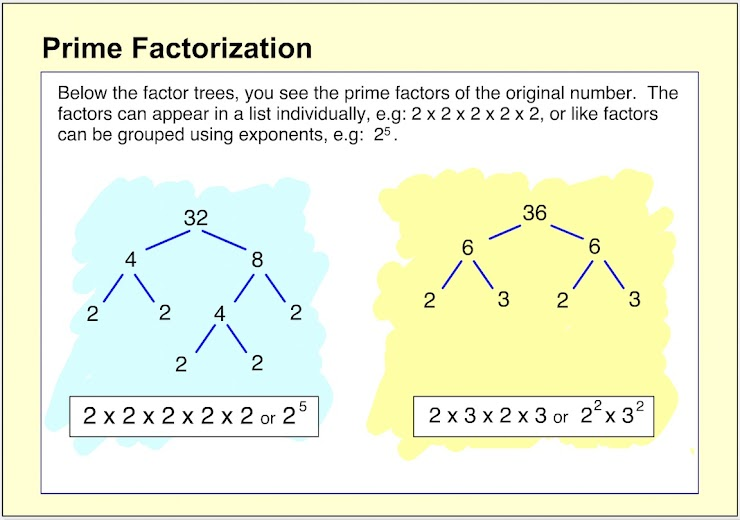Prime Factorization Help Slide 4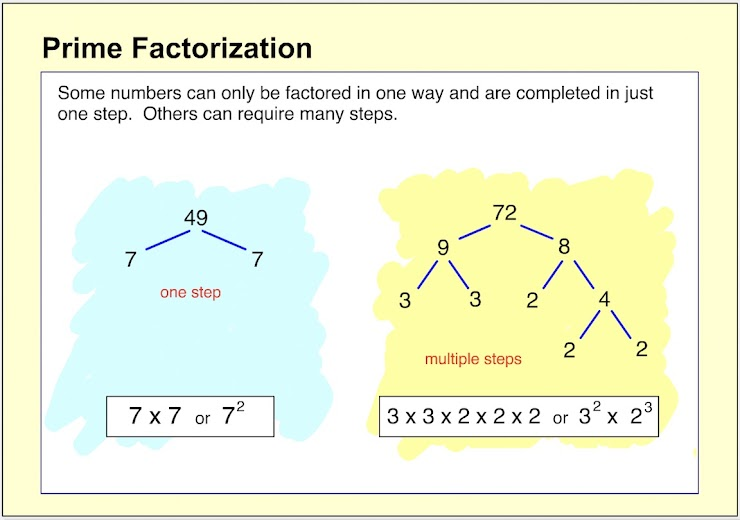Prime Factorization Help Slide 5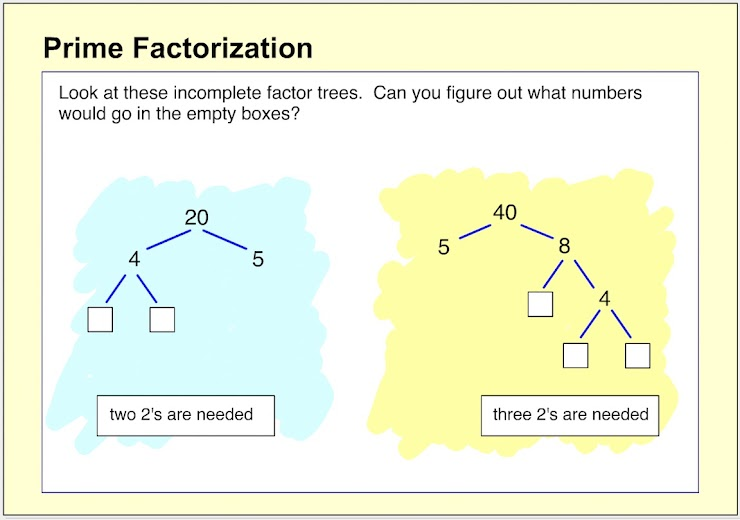1.  What are the prime factors of 20? *
1 point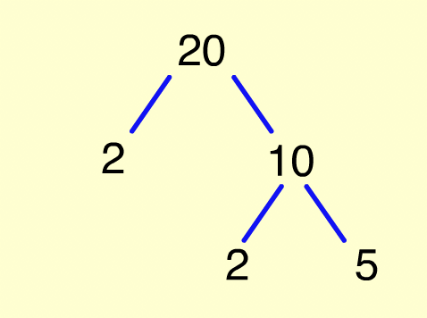2. What are the prime factors of 49? *
1 point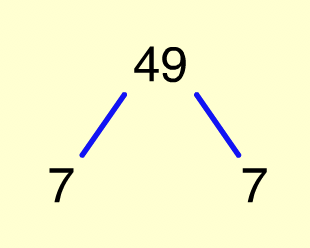3. What are the prime factors of 50? *
1 point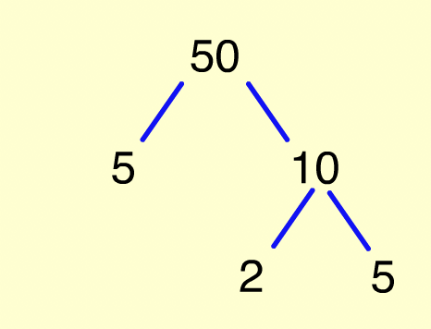4. Which choice shows the prime factors of 28? (Notice the exponents used in some of the answer choices, e.g: 2², 7². Refer back to the Help slides above for more information.) *
1 point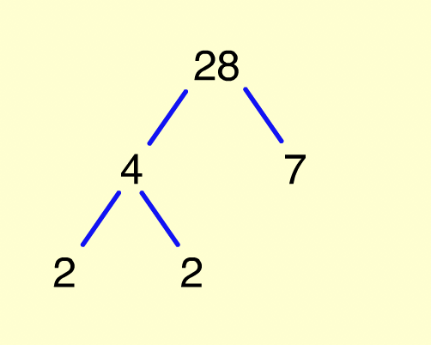5.  Which choice shows the prime factors of 44? *
1 point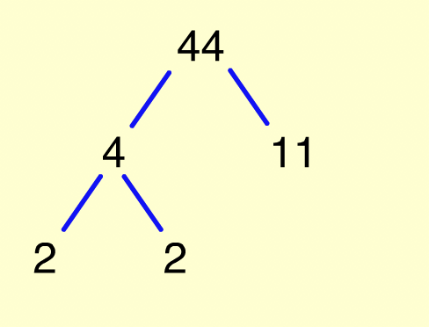6.  Which choice shows the prime factors of 100? *
1 point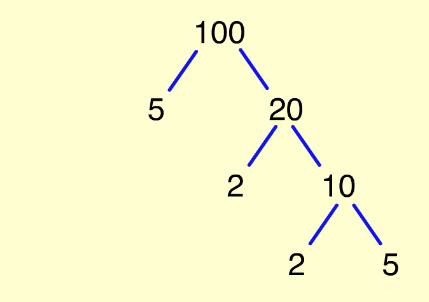7.  What exponents (A and B) would be used to complete the prime factorization for 72? *
1 point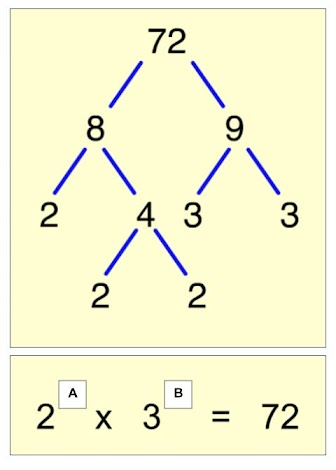8.  What exponent (A) would be used to complete the prime factorization for 84? *
1 point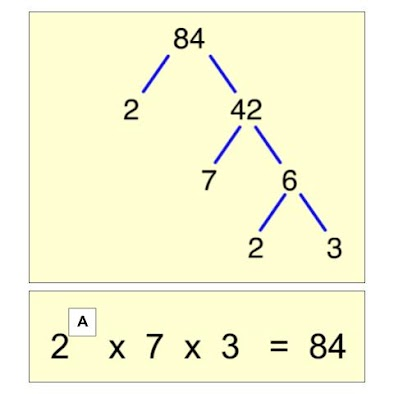9.  True or false? While may numbers can be factored in different ways, the prime factors of the original number will always come out the same. *
1 point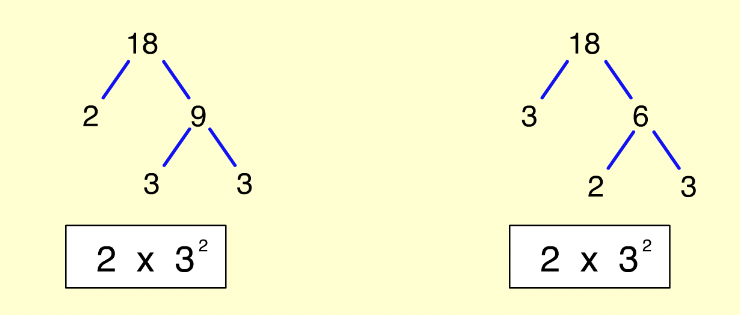10.  What exponent would go in the white box to complete the prime factorization of 81? *
1 point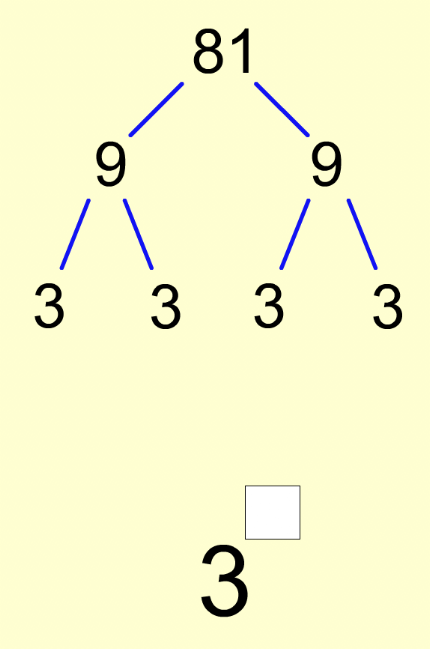Submit
Clear form